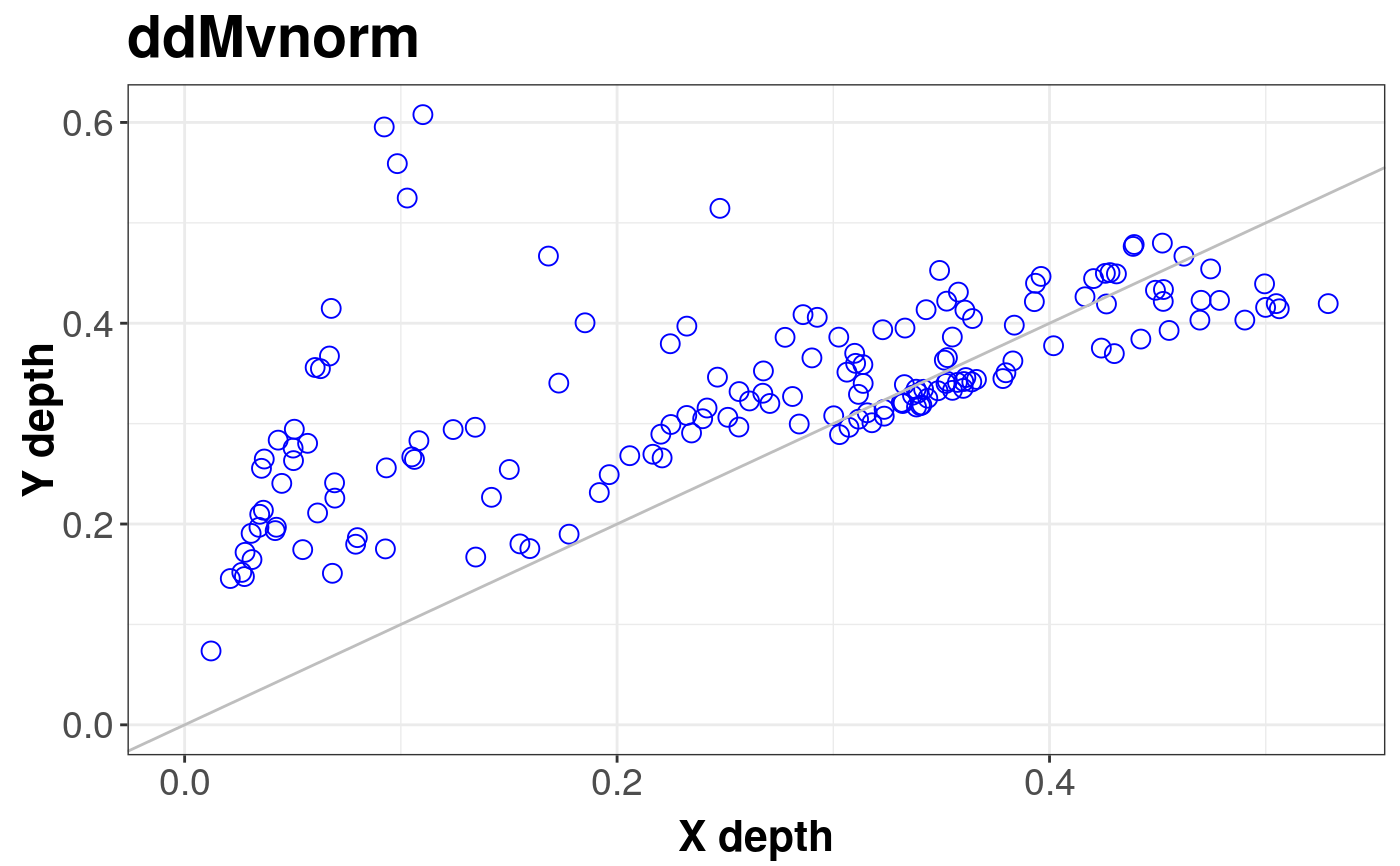Produces a normal DD plot of a multivariate dataset.

ddMvnorm(
x,
size = nrow(x),
robust = FALSE,
alpha = 0.05,
title = "ddMvnorm",
depth_params = list()
)

## Arguments

x The data sample for DD plot. size of theoretical set Logical. Default FALSE. If TRUE, robust measures are used to specify the parameters of theoretical distribution. cutoff point for robust measure of covariance. title of a plot. list of parameters for function depth (method, threads, ndir, la, lb, pdim, mean, cov, exact).

## Value

Returns the normal depth versus depth plot of multivariate dataset x.

## Details

In the first step the location and scale of x are estimated and theoretical sample from normal distribution with those parameters is generated. The plot presents the depth of empirical points with respect to dataset x and with respect to the theoretical sample.

Liu, R.Y., Parelius, J.M. and Singh, K. (1999), Multivariate analysis by data depth: Descriptive statistics, graphics and inference (with discussion), Ann. Statist., 27, 783--858.

Liu, R.Y., Singh K. (1993), A Quality Index Based on Data Depth and Multivariate Rank Test, Journal of the American Statistical Association vol. 88.

ddPlot to generate ddPlot to compare to datasets or to compare a dataset with other distributions.

## Examples

# EXAMPLE 1
norm <- mvrnorm(1000, c(0, 0, 0), diag(3))
con <- mvrnorm(100, c(1, 2, 5), 3 * diag(3))
sample <- rbind(norm, con)
ddMvnorm(sample, robust = TRUE)#> DDPlot#>
#> Depth Metohod:
#> 	 Projection
# EXAMPLE 2
data(under5.mort, inf.mort, maesles.imm)
data1990 <- na.omit(cbind(under5.mort[, 1], inf.mort[, 1], maesles.imm[, 1]))
ddMvnorm(data1990, robust = FALSE)#> DDPlot#>
#> Depth Metohod:
#> 	 Projection